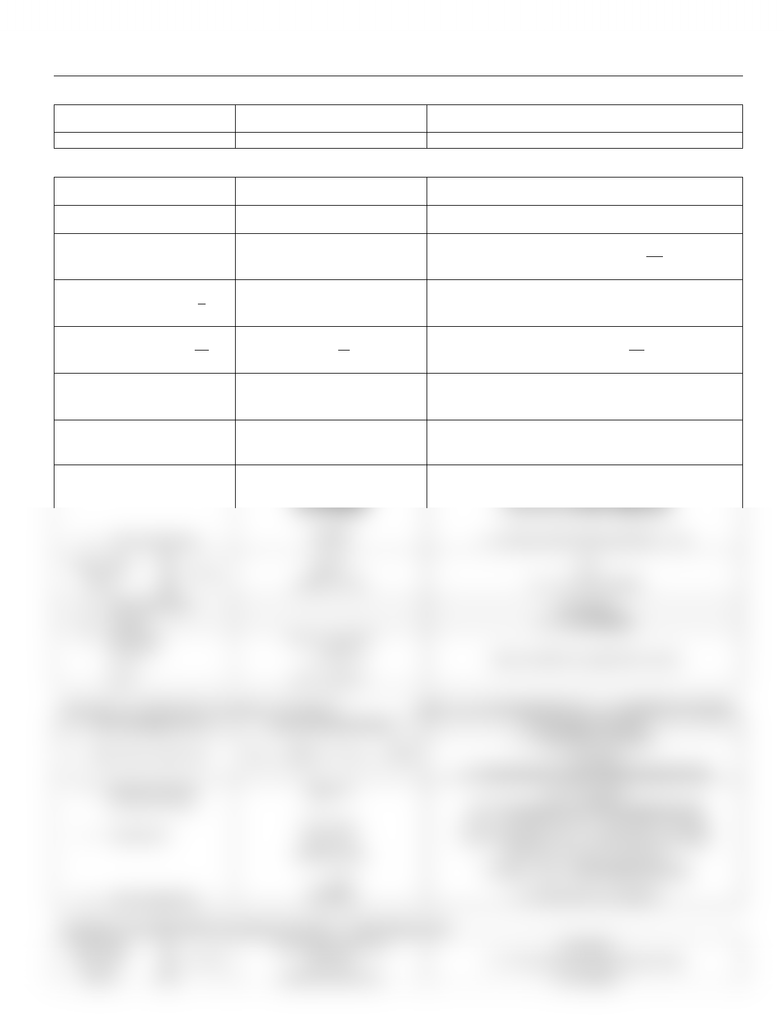Study Guides (380,000)
CA (150,000)
Western (10,000)
CALC (100)
Midterm

Calculus 1301A/B Study Guide - Midterm Guide: Alternating Series Test, Alternating Series, Direct Comparison Test

Department
Calculus
Course Code
CALC 1301A/B
Professor
Natalia Kiriushcheva
Study Guide
Midterm

This preview shows half of the first page. to view the full 2 pages of the document.Summary and Strategies for Convergent Tests
Type
Strategy
Condition for Convergency
Sequences (function)
an


Converges at existing limit (each term approaches it)
rn
|r| < 1
Sum
Sn


Converges at existing limit (sum of series = limit)
Telescoping


Converges at existing limit
Geometric
Series
rn = (r)rn-1
|r| < 1 converging at  

Harmonic
Series
Diverges
P Series
n-p =
p > 1 converging at

General


≠ 0 divergent
= 0 inconclusive, use the following tests
Integral

(easy to integrate)
Positive and decreasing at [a,∞)
Finite number
Comparison
Limit Comparison
≤ bn
≥ bn
positive series


If bn converges through other tests, then converges
If bn diverges through other tests, then diverges
If test fails, use limit comparison
L > 0 (since series always positive, L > 0)
Alternating
Series


cos(n) (-1)n
= 0
an+1 < an (decreasing)
Harmonic Series
Convergent
P series
p > 0 (1 included)
Geometric
(-1)nr(n-1)
(-1)nrn
(-1)n = (-1)(-1)(n-1)
= (-)(-r)(n-1)
(-r)n = (-r)(-r)n-1
Same conditions as geometric series
Absolutely or Conditionally Convergent or Divergent? (If 
 is convergent then
 is absolutely convergent)
1. Test convergency of
Use any methods above
If convergent, proceed
2. Ratio Test or Root Test
 
or  
L < 1 absolutely convergent
L > 1 divergent
L = 1 inconclusive, use the absolute general test
Absolute General
(or other sum test)
Comparison
Limit comparison

 
 ≤ |bn|
 |bn|
Positive series


≠ 0 divergent
= 0 inconclusive, use the comparison test
If |bn| converges, then absolutely converges
If |bn| diverges, then conditionally converges
If test fails, use limit comparison
* (-1)n alternating series test
L > 0 (absolutely convergent)
Examples of Conditionally Convergent Functions alternating series
Alternating
Harmonic
Series


Alternating series test
Root test
Absolute value test
= divergent
= 1 inconclusive (use absolute test)
= convergent
You're Reading a Preview

Unlock to view full version